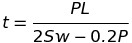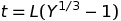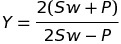# How To: Determine The Thickness Of A Blank Unstayed Full-Hemispherical Head

When completing ASME code calculations involving cylindrical components it is possible during examinations you will be required to know how to determine the thickness of a blank unstayed full-hemispherical head. I recommend before working on questions involving hemispherical head questions you read over and complete the practice questions regarding How To: Determine The Thickness Of A Blank Unstayed Dished Head.

Throughout this post I will provide and formula(s) and where to find them. Describe the variables used in the formula(s). Provide insight or sketches to help describe any of the code requirements I have found particularly important for understanding blank unstayed full-hemispherical head questions. Then provide some completed practice questions to help reinforce the material before wrapping everything up with a summary of the topic.

All reference page numbers are from the 2007 ASME Boiler & Pressure Vessel Code

## Formula

The formula for how to determine the thickness of a blank unstayed full-hemispherical head with pressure on the concave side is under PG-29.11 page 14.

Minimum Thickness FormulaThe above equation should not be used when the required thickness of the head given by the above formula exceeds 35.6% of the inside radius, and instead these following two equations should be used:Where## Formula Variables

The formula variables above are explained on page 14 and are as follows:

L = Is the radius to which the head was formed, measures on the concave side of the head

P = Maximum allowable working pressure

S = Maximum allowable working stress, using values given in Table 1A of Section II, Part D

t = Minimum thickness of head

w = weld joint strength reduction factor per PG-26

## Important Full-Hemispherical Head Code Requirements

PG-29.12 page 14 – If a flanged-in manhole that meets the code requirements is placed in a full-hemispherical head, the thickness of the head shall be the same as for a head dished to a segment of a sphere ( see PG-29.1 and PG -29.5), with a dish radius equal to eight tenths the diameter of the shell and with the added thickness for the manhole as specified in PG-29.3.

[table id=1 /]

## How To: Determine The Thickness Of A Blank Unstayed Full-Hemispherical Head Questions

Note: All code questions are to be calculated in (mm) and (MPa) unless otherwise stated. Convert accordingly and properly before the calculation.

## Summary: Determine The Thickness Of A Blank Unstayed Full-Hemispherical Head

Thanks,

Power Engineering 101

### 1 thought on “How To: Determine The Thickness Of A Blank Unstayed Full-Hemispherical Head”

1.Hi, sir:
I have a different understanding or solving way for Q23.
I think that the equation PG-29.1(according to PG-29.12)should be used. From equation 29.1 calculation, we can get the thickness of the header. Then PG-29.5 is used to get L (the head with manhole), which equals 2×285+2t. Finally, equation PG-29.3 should be applied(15%) to get the final thickness with a manhole.

The method #2: Directly apply equation PG-29.1, where L=(2×285+2t)x0.8, then final thickness plus 15%.

Moreover, there is an error in Q23. The manhole diamention should be less than 380 mm. So, the 127 mm doesn’t meet the code.